## LDBN

This html bundle contains all the data that was collected while performing tests and comparisons between the LDBN sampler and various other samplers from the state of the art. You can click on any sampler below to see all its associated data, discrepancy results, integration variance results, point distribution, behaviour relatively to aliasing (zoneplate tests) and spectral behaviour. Click on point set to get the information.

Back to project website

LDBN
 m=1 t=64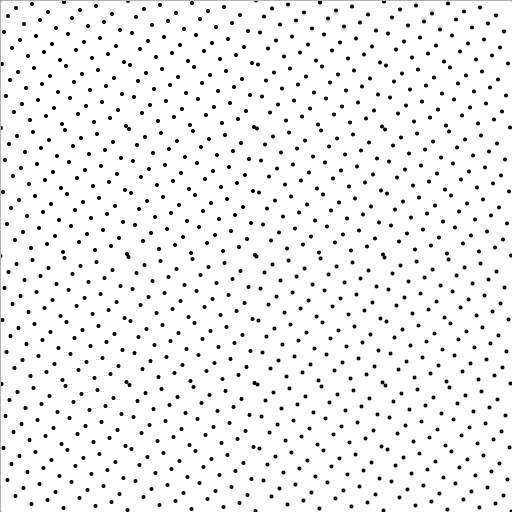m=4 t=64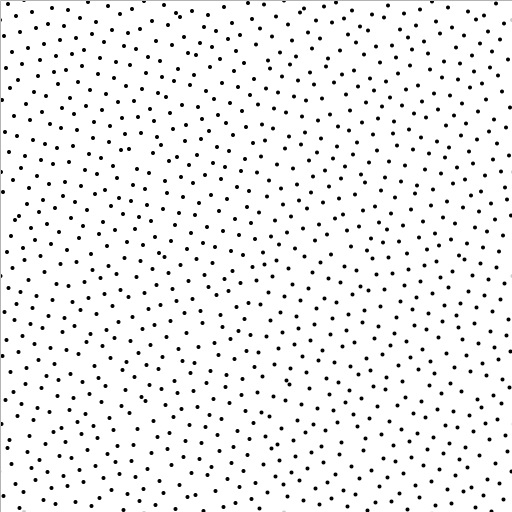m=16 t=64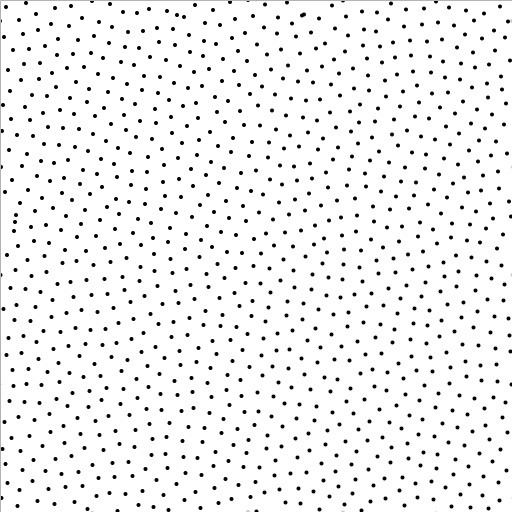m=64 t=64m=1 t=128m=4 t=128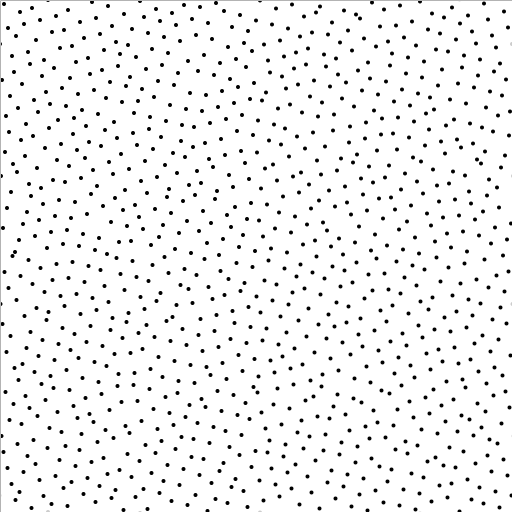m=16 t=128m=64 t=128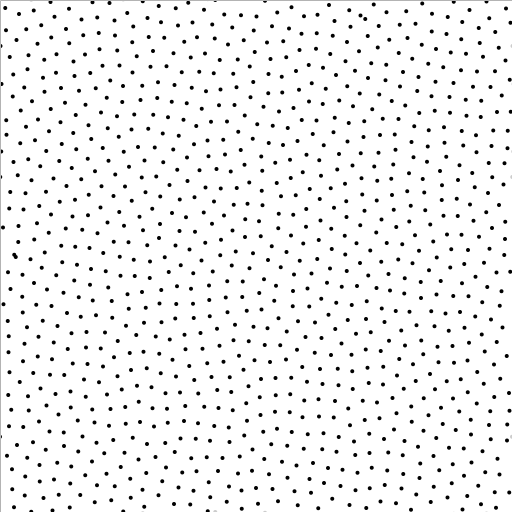m=1 t=256m=4 t=256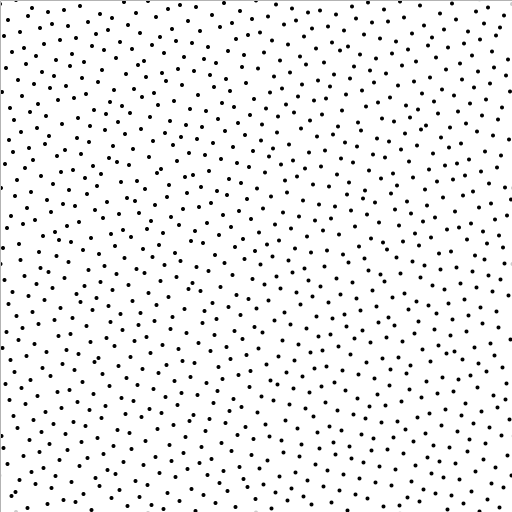m=16 t=256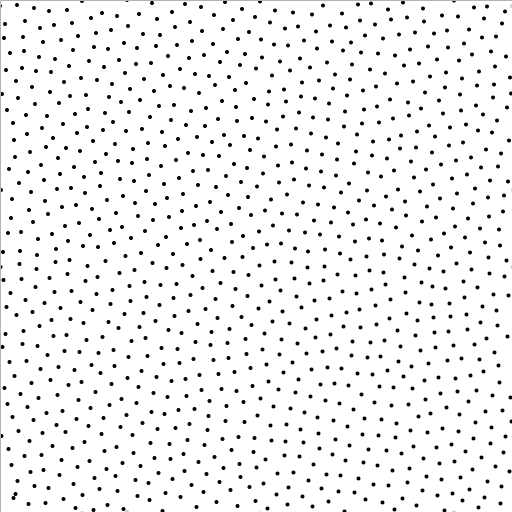m=64 t=256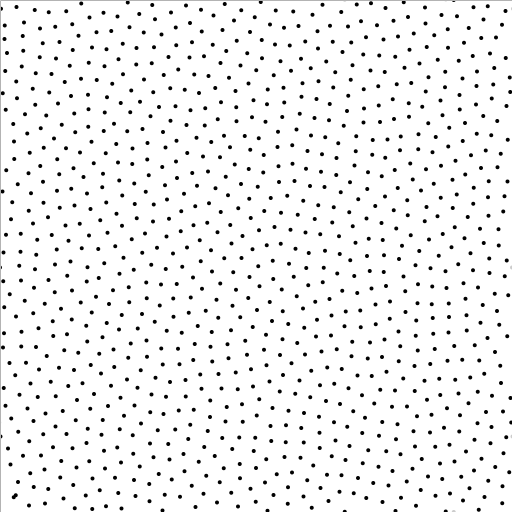Blue Noise Samplers
 BNOT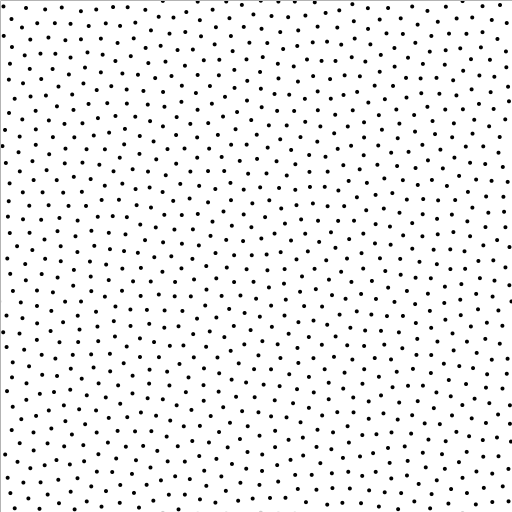CapCVTCVT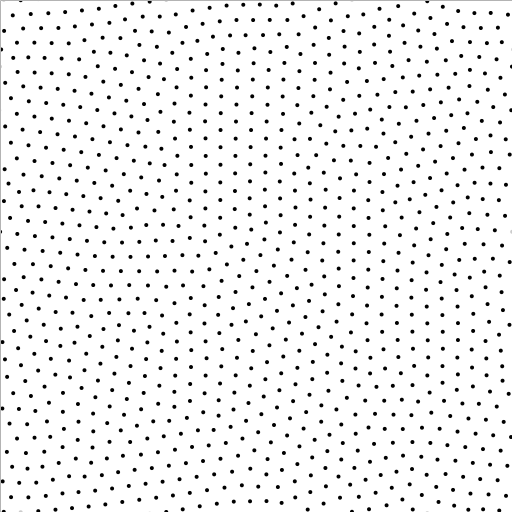AA PatternFPOPolyhexes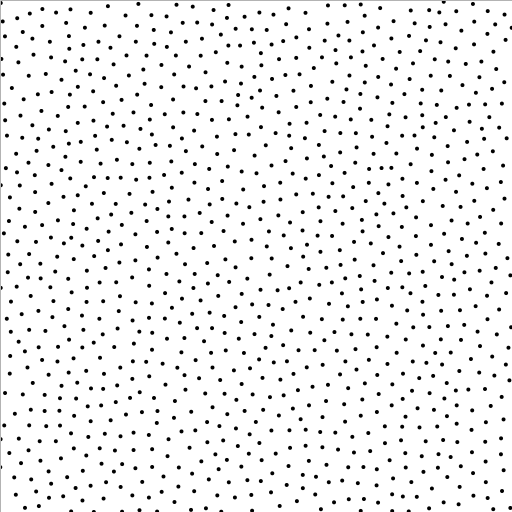Wang TilesPoissonLow Discrepancy Samplers
 Faure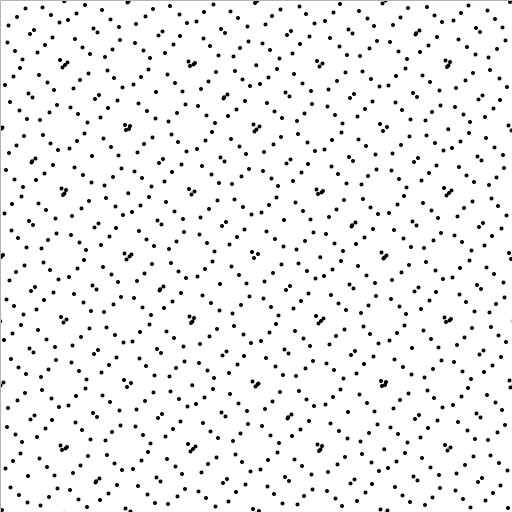Halton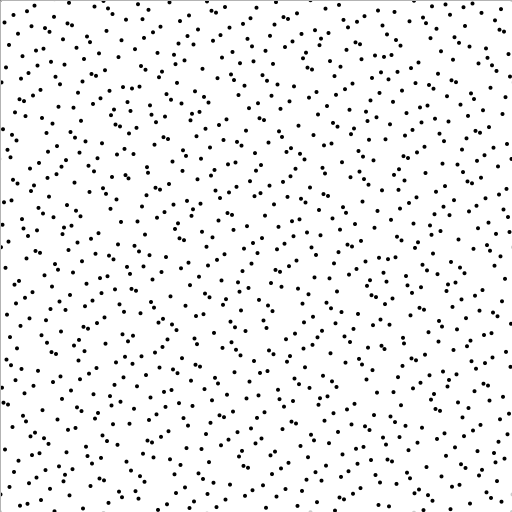HammersleyNiederreiter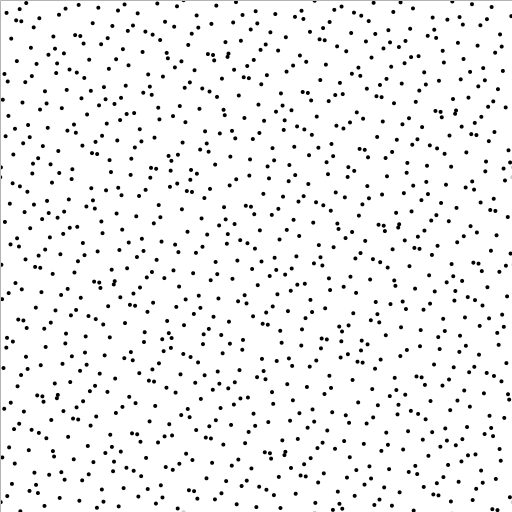Sobol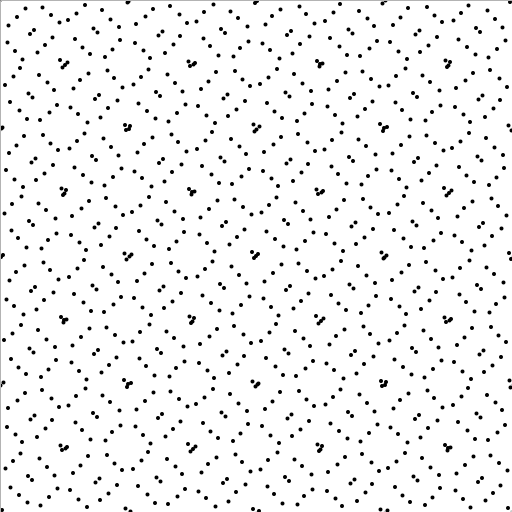Owen's scrambling over Sobol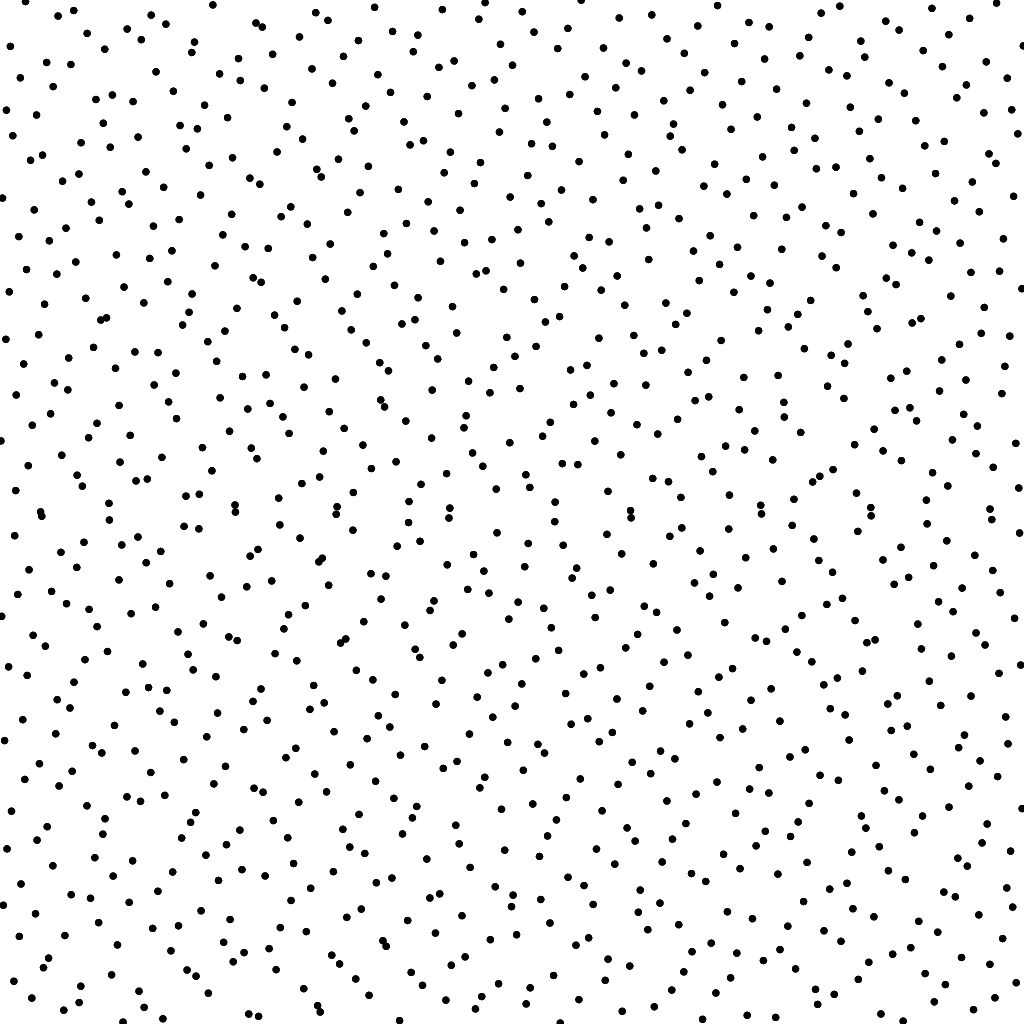Other Samplers
 CMJ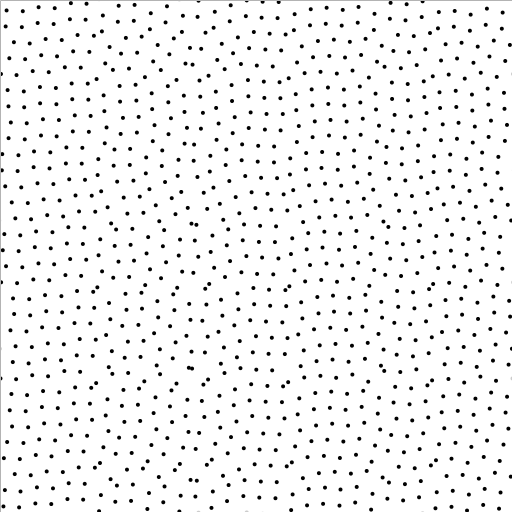HexagonalGridNRooks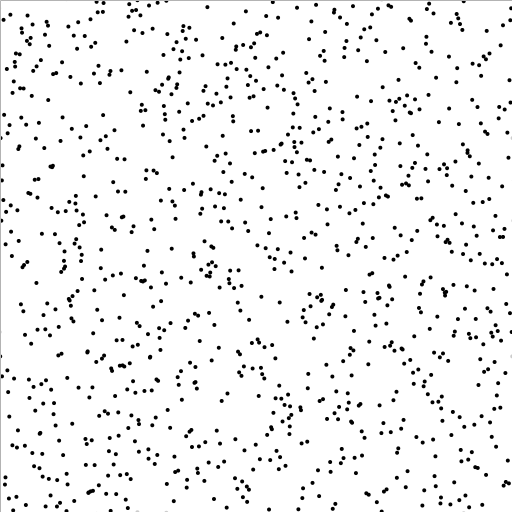Rank 1Rank 1 FibonnacciStep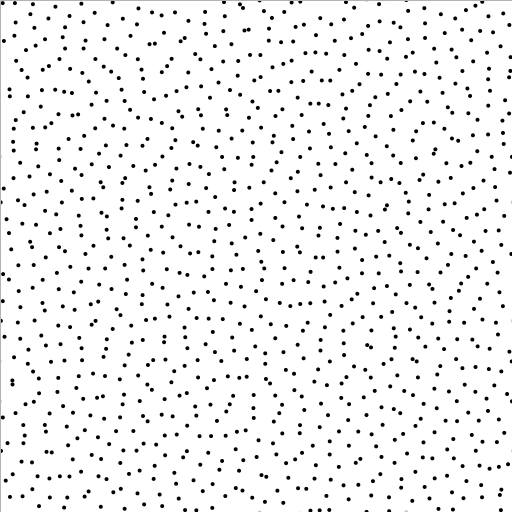Jittered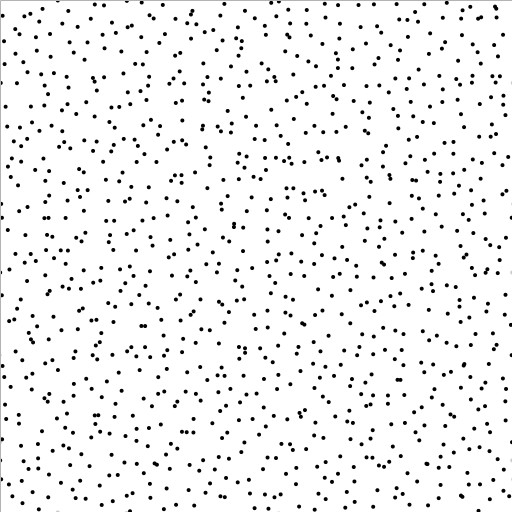WhitenoiseRegularGrid